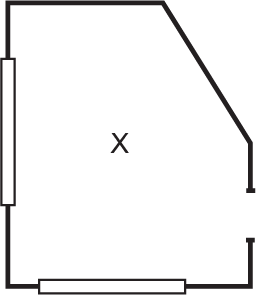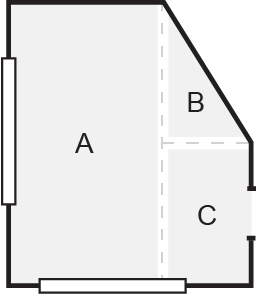# Measuring Guide

In order to determine the square footage of flooring you’ll need, let’s flashback (very briefly!) to high school geometry class.

Your space can be divided into groups of rectangles. All you have to do is calculate the length and width of each of these rectangles, add them together, and you’ll have the total amount flooring you’ll need.

## Here's an Example

Let's say your room looks like "X"Simply break the space into several rectangles and triangles like “A“, “B“, and “C“#### How do I find the area of a rectangle?

Multiply the base by the height.#### How do I find the area of a triangle?

Multiply the base by the height, and then divide by 2..

Measure the length and width of each of these sections, use the proper equation to find the area for each section measured, add them all up to get the total square footage of the space.X = A + B + C

A = 60 x 25 = 1,500sq ft

B = (30 x 15) ÷ 2 = 225sq ft

C = 30 x 15 = 450sq ft

X = 2,175sq ft

Once you have the total square footage of your space you need to add an additional 10% to get the amount of flooring you need (we calculate this for you on our product page). This is critical for a number of reasons, such as if you make cuts and can’t use an entire board, boards get damaged during installation (it happens more than you’d think), any repairs that need to be made, as well as a safety net for any measuring errors. Running short is a costly and time consuming mistake. Having some material left over at the end of the job is a sign you did it right!

Finally, it is important to note that while this is a basic guide, every job requires different specifics and challenges. Things like the thickness of the floor, the clearance of the cabinets, and door jams, etc. may need to be accounted for. It is wise to have a professional check your work, and make sure you order what you need for your specific job.

Questions? We’re happy to walk you through it.